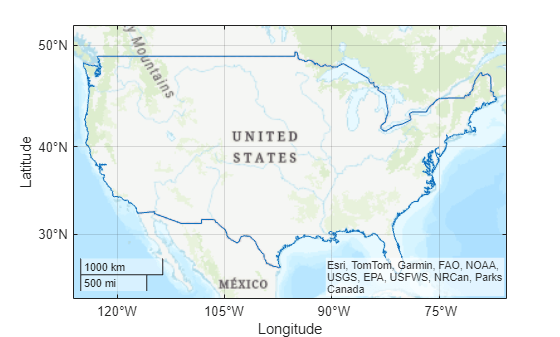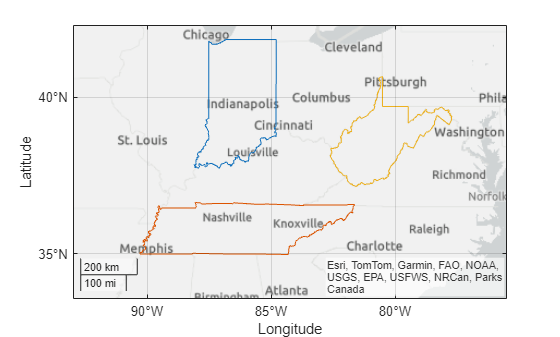# geoplot

Plot line in geographic coordinates

## Syntax

``geoplot(lat,lon)``
``geoplot(lat,lon,LineSpec)``
``geoplot(lat1,lon1,...,latN,lonN)``
``geoplot(lat1,lon1,LineSpec1,...,latN,lonN,LineSpecN)``
``geoplot(___,Name,Value)``
``geoplot(gx,___)``
``h = geoplot(___)``

## Description

example

````geoplot(lat,lon)` plots a line in a geographic axes with vertices at the latitude-longitude locations specified (in degrees) by the vectors `lat` and `lon`. The `lat` and `lon` inputs must be the same size. By default, `geoplot` draws a solid line, with no markers, using colors specified by the `ColorOrder` property of the Geographic axes object.```

example

````geoplot(lat,lon,LineSpec)` sets the style, marker symbol, and color for the line.```
````geoplot(lat1,lon1,...,latN,lonN)` combines the plots specified by several sets of latitude-longitude locations.```

example

````geoplot(lat1,lon1,LineSpec1,...,latN,lonN,LineSpecN)` combines the plots specified by several sets of latitude-longitude locations, with a separate `LineSpec` for each line.```

example

````geoplot(___,Name,Value)` specifies properties of the chart line using one or more `Name,Value` pair arguments.```
````geoplot(gx,___)` plots in the geographic axes specified by `gx` instead of the current axes. ```
````h = geoplot(___)` returns a column vector of Chart Line objects. Each object corresponds to a plotted line. Use `h` to modify the properties of the Chart line objects.```

## Examples

collapse all

Plot a straight line between two points on a map. Specify the endpoints of the line using the coordinates of Seattle and Anchorage. Specify latitude and longitude in degrees.

```latSeattle = 47.62; lonSeattle = -122.33; latAnchorage = 61.20; lonAnchorage = -149.9;```

Plot the data on a map. Customize the appearance of the line using the line specification `'g-*'`. Adjust the latitude and longitude limits of the map using `geolimits`.

```geoplot([latSeattle latAnchorage],[lonSeattle lonAnchorage],'g-*') geolimits([45 62],[-149 -123])```Plot a straight line between two points on a map. Specify the endpoints of the line using the coordinates of Seattle and Anchorage. Specify latitude and longitude in degrees.

```latSeattle = 47.62; lonSeattle = -122.33; latAnchorage = 61.20; lonAnchorage = -149.9;```

Plot the data using `geoplot`. Customize the appearance of the line using the line specification `'g-*'`. Adjust the latitude and longitude limits of the map using `geolimits`.

```geoplot([latSeattle latAnchorage],[lonSeattle lonAnchorage],'g-*') geolimits([45 62],[-149 -123])```Identify Anchorage using the `text` function.

`text(latAnchorage,lonAnchorage,'Anchorage');`Identify Seattle using the `text` function. Adjust the alignment of the text label by specifying properties of the `Text` object.

```text(latSeattle,lonSeattle,'Seattle',... 'HorizontalAlignment','right',... 'VerticalAlignment','bottom');```Specify the latitude and longitude coordinates of Seattle, Anchorage, and Point Barrow.

```latSeattle = 47.62; lonSeattle = -122.33; latAnchorage = 61.20; lonAnchorage = -149.9; latPtBarrow = 71.38; lonPtBarrow = -156.47;```

Plot straight lines from Seattle to each of the other two cities. Draw a solid yellow line from Seattle to Anchorage and a dotted blue line between Seattle and Point Barrow. Adjust the latitude and longitude limits of the map using `geolimits`.

```geoplot([latSeattle latAnchorage],[lonSeattle lonAnchorage],'y-',... [latSeattle latPtBarrow],[lonSeattle lonPtBarrow],'b:') geolimits([44 73],[-149 -123])```Label each city on the map using the `text` function.

```text(latAnchorage,lonAnchorage,'Anchorage'); text(latPtBarrow,lonPtBarrow,'Point Barrow'); text(latSeattle,lonSeattle,'Seattle',... 'VerticalAlignment','bottom');```Plot a straight line between two points on a map. Specify the endpoints of the line using the coordinates of Seattle and Anchorage. Specify latitude and longitude in degrees.

```latSeattle = 47.62; lonSeattle = -122.33; latAnchorage = 61.20; lonAnchorage = -149.9;```

Plot the data using `geoplot`. Adjust the latitude and longitude limits of the map using `geolimits`. Customize the appearance of the line by specifying the `LineWidth` and `Color` properties.

```geoplot([latSeattle latAnchorage],[lonSeattle lonAnchorage],... 'LineWidth',2,'Color',[.6 0 0]) geolimits([45 62],[-149 -123])```Plot a straight line between two points on a map. Specify the endpoints of the line using the coordinates of Seattle and Anchorage. Specify latitude and longitude in degrees.

```latSeattle = 47.62; lonSeattle = -122.33; latAnchorage = 61.20; lonAnchorage = -149.9;```

Plot the data using `geoplot`. Adjust the latitude and longitude limits of the map using `geolimits`.

```geoplot([latSeattle latAnchorage],[lonSeattle lonAnchorage]) geolimits([45 62],[-149 -123])```Change the basemap used in the plot using the `geobasemap` function.

`geobasemap colorterrain`## Input Arguments

collapse all

Latitude coordinates in degrees, specified as a real, numeric, finite vector. The vector can contain embedded `NaN`s. `lat` must be the same size as `lon`.

Example: `[43.0327 38.8921 44.0435]`

Data Types: `single` | `double`

Longitude coordinates in degrees, specified as a real, numeric, finite vector. The vector can contain embedded `NaN`s. `lon` must be the same size as `lat`.

Example: `[-107.5556 -77.0269 -72.5565]`

Data Types: `single` | `double`

Line specification, specified as a character vector or string containing a line style, marker, and color specifier. The elements can appear in any order, and you can omit one or more options. If you omit the line style and specify the marker, the plot shows only markers with no line connecting them. For more information, see ```LineSpec (Line Specification)```.

Example: `'--or'` is a red dashed line with circular markers

Data Types: `char` | `string`

Target geographic axes, specified as a `GeographicAxes` object. You can modify the appearance and behavior of a `GeographicAxes` object by setting its properties. For a list of properties, see GeographicAxes Properties.

### Name-Value Pair Arguments

Specify optional comma-separated pairs of `Name,Value` arguments. `Name` is the argument name and `Value` is the corresponding value. `Name` must appear inside quotes. You can specify several name and value pair arguments in any order as `Name1,Value1,...,NameN,ValueN`.

Example: `h = geoplot(lat,lon,'Color','g')`

### Note

Property settings apply to all the lines in the plot. To set the properties of an individual line, retrieve the handle to the line in the `geoplot` return value `h` and use dot notation.

The name-value pairs listed here are only a subset. For a full list, see Line Properties.

Line color, specified as a MATLAB ```ColorSpec (Color Specification)```. If specifying an RGB triplet, the intensities must be in the range `[0,1]`.

Example: `'green'`

Example: `'g'`

Example: `[0 1 0]`

Line style, specified as a MATLAB ```LineSpec (Line Specification)```.

Example: `'--'` (dashed line)

Example: `':'` (dotted line)

Line width, specified as a positive value in points, where 1 point = 1/72 of an inch. If the line has markers, then the line width also affects the marker edges.

The line width cannot be thinner than the width of a pixel. If you set the line width to a value that is less than the width of a pixel on your system, the line displays as one pixel wide.

Marker symbol, specified as one of the markers in this table. By default, a chart line does not have markers. Add markers at each data point along the line by specifying a marker symbol.

ValueDescription
`'o'`Circle
`'+'`Plus sign
`'*'`Asterisk
`'.'`Point
`'x'`Cross
`'square'` or `'s'`Square
`'diamond'` or `'d'`Diamond
`'^'`Upward-pointing triangle
`'v'`Downward-pointing triangle
`'>'`Right-pointing triangle
`'<'`Left-pointing triangle
`'pentagram'` or `'p'`Five-pointed star (pentagram)
`'hexagram'` or `'h'`Six-pointed star (hexagram)
`'none'`No markers

Marker face color, specified as `'none'`, `'auto'` or a MATLAB ```ColorSpec (Color Specification)```. The `'auto'` value uses the same color as the `Color` name-value pair for the axes. If you specify an RGB triplet, the intensities must be in the range `[0,1]`.

Example: `'green'`

Example: `'g'`

Example: `[0 1 0]`

Marker size, specified as a positive value in points, where 1 point = 1/72 of an inch.

## Output Arguments

collapse all

Geographic plot, returned as a column vector of `Line` objects. Each object corresponds to a plotted line. Use `h` to modify the properties of the objects after they area created.

## Tips

• Plot 3-D geographic data using the Mapping Toolbox™ functions `geoglobe` and `geoplot3`.

• To customize certain aspects of your plot, use properties of the geographic axes. For a list of properties, see GeographicAxes Properties.

• To plot additional data in the geographic axes, use the ```hold on``` command.

• You cannot plot data that requires Cartesian axes in a geographic axes.